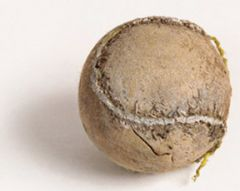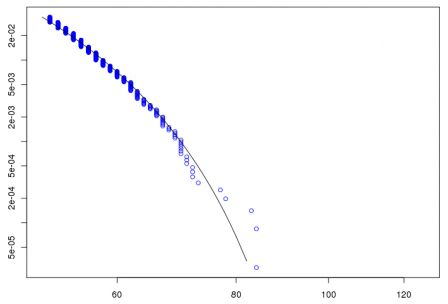Want to share your content on R-bloggers? click here if you have a blog, or here if you don't.

As mentioned already here, while we were going to Québec City for the workshop, we had interesting discussions in the car, and Maciej mentioned an article recently published in The Actuary,Hence, I wanted to discuss (extremely) rare event probabilities in tennis. The story is simple: in June 2010, at Wimbledon, Nicolas Mahut and John Isner have played the longest match ever. 980 points, 11 But first of all, we need a dataset. Thanks to Duncan Murdoch, I have been able to run a short code to build up a dataset:

```CITIES=c("berlin","madrid","paris","rolandgarros","wimbledon","sydney",
"beijing","shanghai","singapore","tokyo","melbourne","melbourne-indoor")
YEARS=1970:2009
BASE0=data.frame(YEAR=NA,TRNMT=NA,LENGTH=NA,SETS=NA)
for(i in 1:length(CITIES)){
for(j in 1:length(YEARS)){
city=CITIES[i]
year=YEARS[j]
localization = paste("http://www.resultsfromtennis.com/",
year,"/atp/",city,".html",sep="")
ERROR404=FALSE
if(inherits(essai, "try-error")){ERROR404=TRUE}
if(ERROR404==FALSE){
B=scan(localization,"character")
SETS=NA
LENGTH=NA
if(length(B)>270){
I=(substr(B,1,10)=="class=rez>")
sum(I)
X0=B[I]
X3=as.numeric(substr(X0,11,13))
X2=as.numeric(substr(X0,11,12))
X1=as.numeric(substr(X0,11,11))
X0=X3
X0[is.na(X3)==TRUE]=X2[is.na(X3)==TRUE]
X0[is.na(X2)==TRUE]=X1[is.na(X2)==TRUE]
JL=c(which(substr(B,1,9)=="class=nl>"),length(B))
IL=which(substr(B,1,10)=="class=rez>")
IC=cut(IL,JL)
base=data.frame(IC,X0)
LENGTH=as.numeric(tapply(X0,IC,sum))
SETS=as.numeric(tapply(X0,IC,length))/2}
BASE=data.frame(YEAR=year,TRNMT=city,LENGTH,SETS)
BASE0=rbind(BASE0,BASE)}}}
write.table(BASE0,"BASE-TENNIS-TOTAL.txt")```

Here I consider only tournaments where players have to win 3 sets (and actually more tournaments than those in the code above), and I have something like a bit more than 72,000 matches,

```> I=is.na(TENNIS\$LENGTH)==FALSE
> BT=TENNIS[I,]
> nrow(BT)
 72754
> maxr=function(x){max(x,na.rm=TRUE)}
> T=paste(BT\$TRNMT,BT\$YEAR)
> DUREE=tapply(BT\$SETS,T,maxr)
> LISTE=names(DUREE[DUREE>3])
> BT=BT[T%in%LISTE,]```

so, if we look briefly at matches over 35 years, we have the following boxplot (one boxplot per year),The red line being the epic Isner-Mahut match in June 2010 (4-6, 6-3, 7-6, 6-7, 70-68, i.e. 183 games, here for the score card).

If we study theory (e.g. from Paul Newton and Kamran Aslam), a lot of results can be obtained for the expected value of the number of games, but if we want to study extremely rare events, we should generate Markov chains (with a lot of generation since the probability should be extremely small). But how many ? Consider below matches with more than 50 games,The tail plot (over 50), i.e. the log-log Pareto plot indicates that it will be difficult to study tails,and similarly with the Hill plot (assuming that tails are Pareto type….)Anyway, if we want to study tails, we should consider a threshold high enough. For instance, with a threshold at 68 (we keep only 24 match), we have

```> seuil=68+0.25
> GPD1=gpd(X,seuil,method = "ml")
> GPD2=gpd(X,seuil,method = "pwm")
>
> xi=GPD1\$par.ests
> mu=seuil
> beta=GPD1\$par.ests
> x=180
> P=exp((-1/xi)*log(1 + (xi * (x - mu))/beta))
> as.numeric((1-GPD1\$p.less.thresh)*P)
 5.621281e-09
>
> xi=GPD2\$par.ests
> mu=seuil
> beta=GPD2\$par.ests
> x=180
> P=exp((-1/xi)*log(1 + (xi * (x - mu))/beta))
> as.numeric((1-GPD2\$p.less.thresh)*P)
 3.027095e-09```

I.e. the probability that one match last more than 183 games is 1 chance over a billion… With, say, 2500 match per year, that gives us a return period of 400 years. So yes, we might say that this way a rare event… So perhaps, generating several billions of chains, it should be possible to get a more precise estimation of the probability to play 183 games in a single match…